login  home  contents  what's new  discussion  bug reports help  links  subscribe  changes  refresh  edit

## Section 9.83 UnivariatePolynomial

The domain constructor UnivariatePolynomial (abbreviated UP) creates domains of univariate polynomials in a specified variable. For example, the domain UP(a1,POLY FRAC INT) provides polynomials in the single variable a1 whose coefficients are general polynomials with rational number coefficients. UP(x,INT) is the domain of polynomials in the single variable x with integer coefficients.

Example operations on univariate polynomials

axiom
(p,q) : UP(x,INT)
Type: Void
axiom
p := (3*x-1)**2 * (2*x + 8)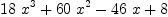(1)
Type: UnivariatePolynomial(x,Integer)
axiom
q := (1 - 6*x + 9*x**2)**2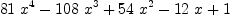(2)
Type: UnivariatePolynomial(x,Integer)
axiom
p**2 + p*q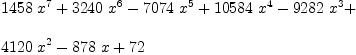(3)
Type: UnivariatePolynomial(x,Integer)
axiom
D p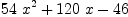(4)
Type: UnivariatePolynomial(x,Integer)
axiom
integrate p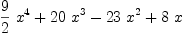(5)
Type: UnivariatePolynomial(x,Fraction(Integer))
axiom
p 2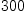(6)
Type: PositiveInteger?
axiom
subst(p,x=2)(7)
Type: Expression(Integer)
axiom
2 p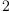(8)
Type: UnivariatePolynomial(x,Integer)
axiom
p q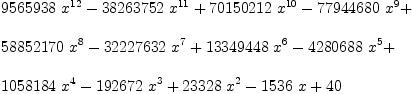(9)
Type: UnivariatePolynomial(x,Integer)
axiom
q p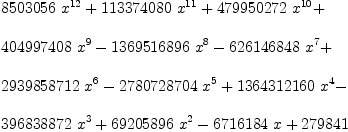(10)
Type: UnivariatePolynomial(x,Integer)
axiom
y:Symbol
Type: Void
axiom
1 y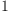(11)
Type: UnivariatePolynomial(y,Integer)
axiom
w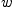(12)
Type: Variable(w)
axiom
p w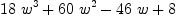(13)
Type: Fraction(Polynomial(Integer))
axiom
vectorise(p,5)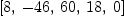(14)
Type: Vector(Integer)
axiom
t : UP(a1,FRAC POLY INT)
Type: Void
axiom
t := a1**2 - a1/b2 + (b1**2-b1)/(b2+3)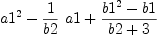(15)
Type: UnivariatePolynomial(a1,Fraction(Polynomial(Integer)))
axiom
u : FRAC POLY INT := t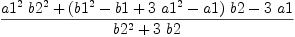(16)
Type: Fraction(Polynomial(Integer))
axiom
u :: UP(b1,?)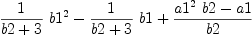(17)
Type: UnivariatePolynomial(b1,Fraction(Polynomial(Integer)))

 Subject:   Be Bold !! ( 14 subscribers )